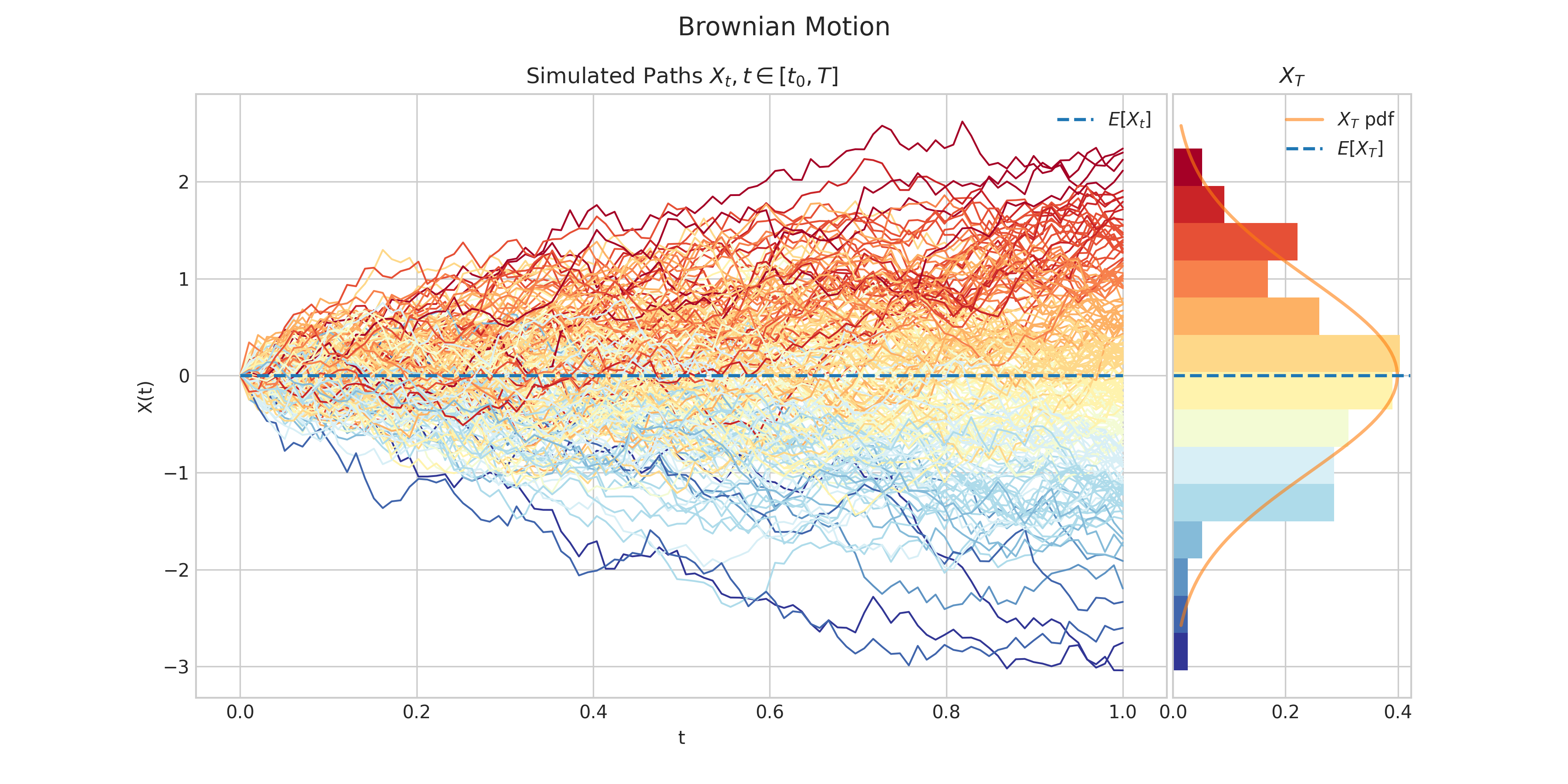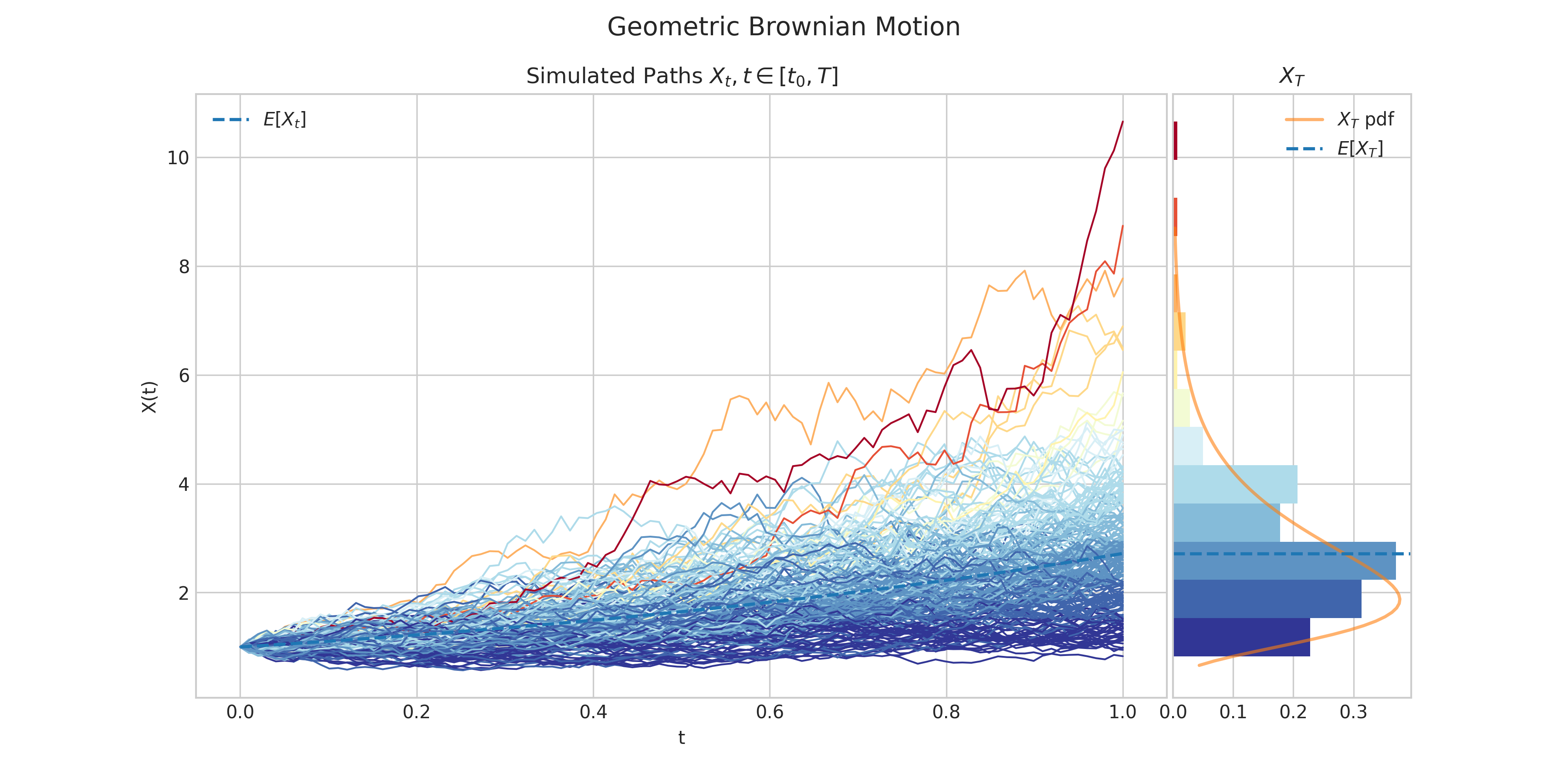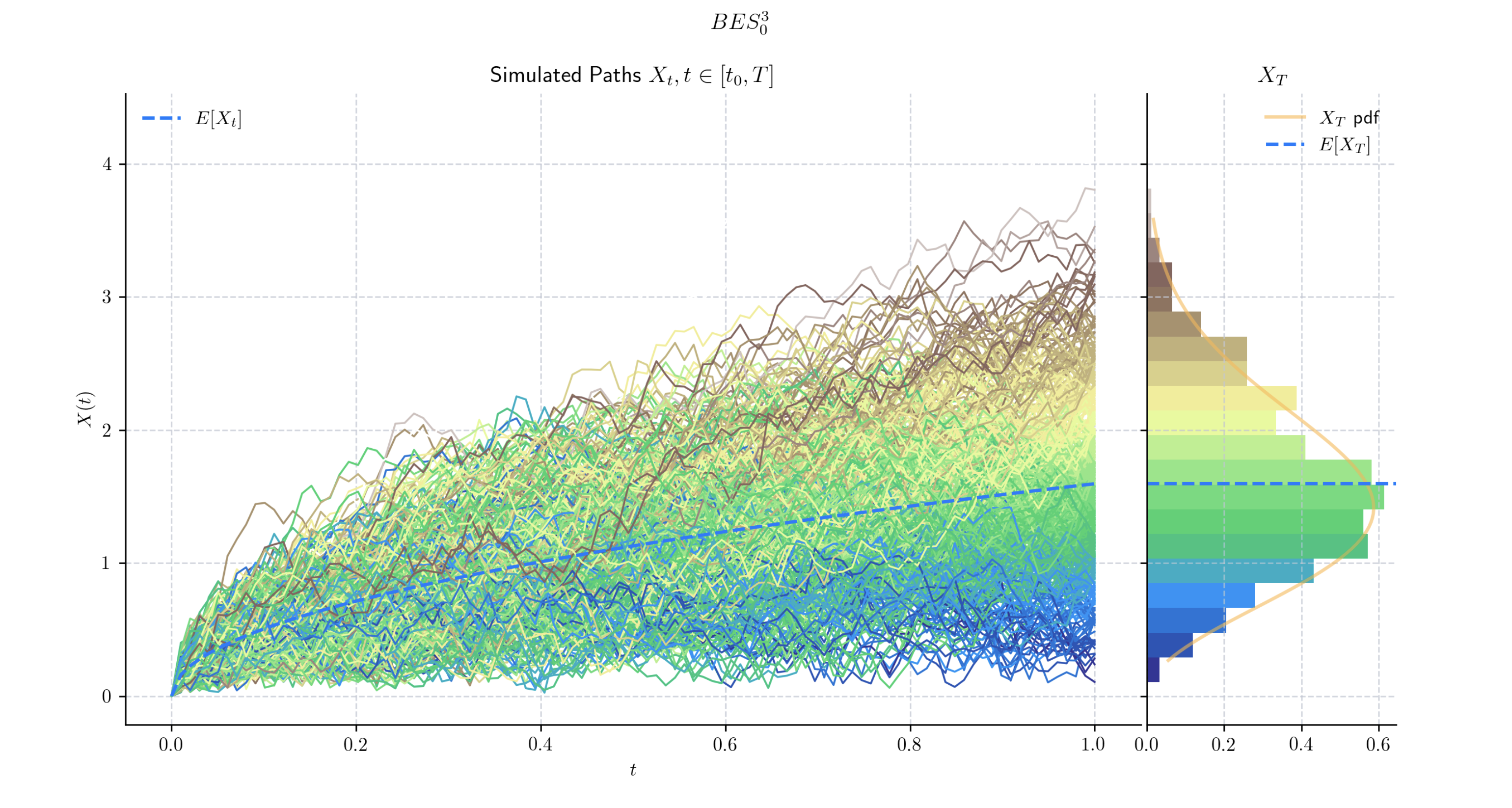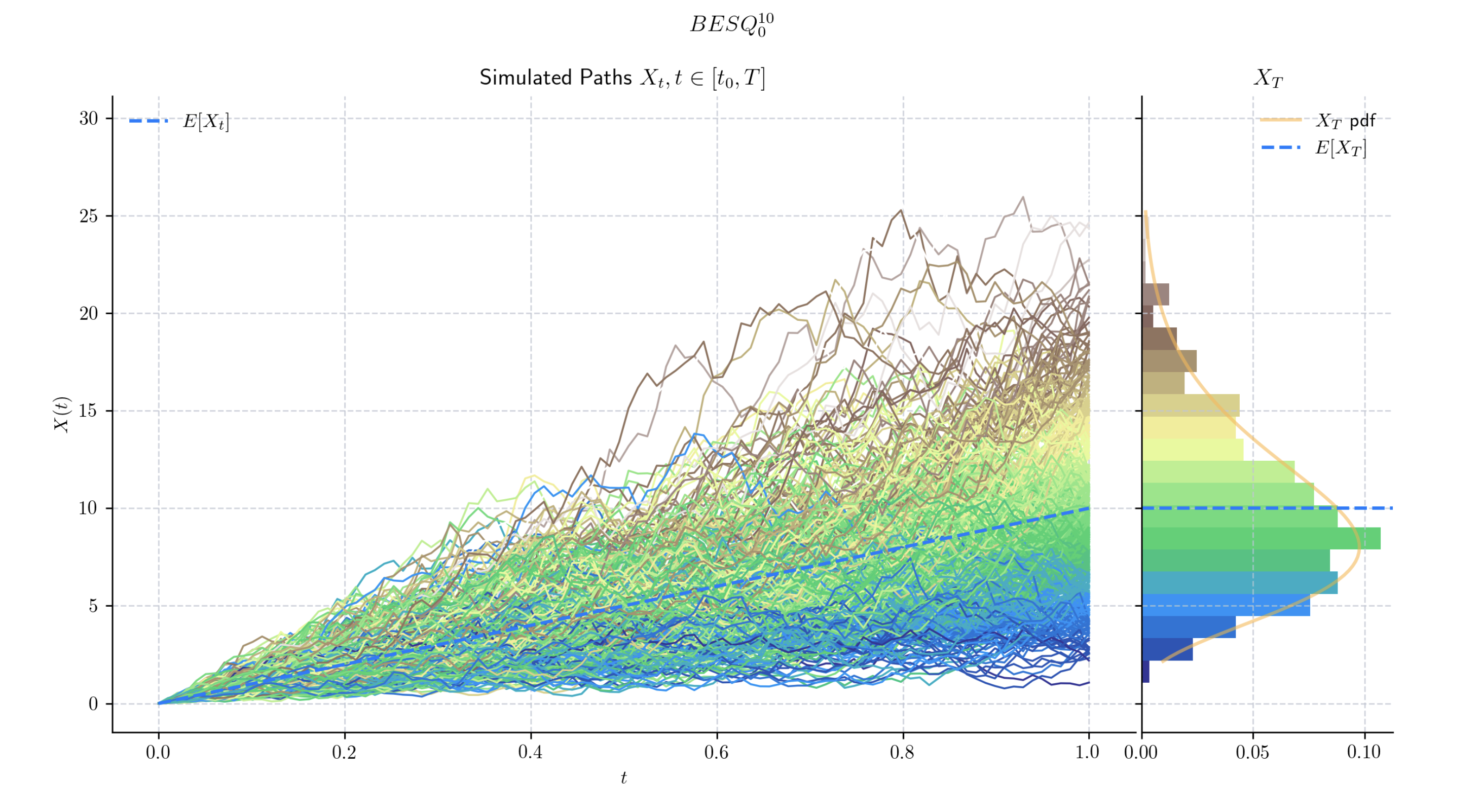# Analytical Processes#

The aleatory.processes module provides classes for continuous-time stochastic processes which can be expressed in analytical form.

class aleatory.processes.BrownianMotion(drift=0.0, scale=1.0, T=1.0, rng=None)[source]#

Brownian motionA standard Brownian motion $$\{W_t : t \geq 0\}$$ is defined by the following properties:

1. Starts at zero, i.e. $$W(0) = 0$$

2. Independent increments

3. $$W(t) - W(s)$$ follows a Gaussian distribution $$N(0, t-s)$$

4. Almost surely continuous

A more general version of a Brownian motion, is the Drifted Brownian Motion which is defined by the following SDE

$dX_t = \mu dt + \sigma dW_t \ \ \ \ t\in (0,T]$

with initial condition $$X_0 = x_0\in\mathbb{R}$$, where

• $$\mu$$ is the drift

• $$\sigma>0$$ is the volatility

• $$W_t$$ is a standard Brownian Motion.

Clearly, the solution to this equation can be written as

$X_t = x_0 + \mu t + \sigma W_t \ \ \ \ t \in [0,T]$

and each $$X_t \sim N(\mu t, \sigma^2 t)$$.

Parameters:
• drift (float) – the parameter $$\mu$$ in the above SDE

• scale (float) – the parameter $$\sigma>0$$ in the above SDE

• initial (float) – the initial condition $$x_0$$ in the above SDE

• T (float) – the right hand endpoint of the time interval $$[0,T]$$ for the process

• rng (numpy.random.Generator) – a custom random number generator

property T#

End time of the process.

draw(n, N, marginal=True, envelope=False, type='3sigma', title=None, **fig_kw)[source]#

Simulates and plots paths/trajectories from the instanced stochastic process.

Produces different kind of visualisation illustrating the following elements:

• times versus process values as lines

• the expectation of the process across time

• histogram showing the empirical marginal distribution $$X_T$$ (optional when marginal = True)

• probability density function of the marginal distribution $$X_T$$ (optional when marginal = True)

• envelope of confidence intervals across time (optional when envelope = True)

Parameters:
• n – number of steps in each path

• N – number of paths to simulate

• marginal – bool, default: True

• envelope – bool, default: False

• type – string, default: ‘3sigma’

• title – string to customise plot title

Returns:

plot(n, N, title=None, **fig_kw)#

Simulates and plots paths/trajectories from the instanced stochastic process. Simple plot of times versus process values as lines and/or markers.

Parameters:
• n – number of steps in each path

• N – number of paths to simulate

• title – string to customise plot title

Returns:

sample(n)[source]#

Generates a discrete time sample from a Brownian Motion instance.

Parameters:

n – the number of steps

Returns:

numpy array

sample_at(times)[source]#

Generates a sample from a Brownian motion at the specified times.

Parameters:

times – the times which define the sample

Returns:

numpy array

simulate(n, N)#

Simulate paths/trajectories from the instanced stochastic process.

Parameters:
• n – number of steps in each path

• N – number of paths to simulate

Returns:

list with N paths (each one is a np.array of size n)

class aleatory.processes.GBM(drift=1.0, volatility=0.5, initial=1.0, T=1.0, rng=None)[source]#

Geometric Brownian MotionA Geometric Brownian Motion $$\{X(t) : t \geq 0\}$$ is characterised by the following SDE.

$dX_t = \mu X_t dt + \sigma X_t dW_t \ \ \ \ t\in (0,T]$

with initial condition $$X_0 = x_0\geq0$$, where

• $$\mu$$ is the drift

• $$\sigma>0$$ is the volatility

• $$W_t$$ is a standard Brownian Motion.

The solution to this equation can be written as

$X_t = x_0\exp\left((\mu + \frac{\sigma^2}{2} )t +\sigma W_t\right)$

and each $$X_t$$ follows a log-normal distribution.

Parameters:
• drift (float) – the parameter $$\mu$$ in the above SDE

• volatility (float) – the parameter $$\sigma>0$$ in the above SDE

• initial (float) – the initial condition $$x_0$$ in the above SDE

• T (float) – the right hand endpoint of the time interval $$[0,T]$$ for the process

• rng (numpy.random.Generator) – a custom random number generator

property T#

End time of the process.

draw(n, N, marginal=True, envelope=False, title=None, **fig_kw)#

Simulates and plots paths/trajectories from the instanced stochastic process. Visualisation shows - times versus process values as lines - the expectation of the process across time - histogram showing the empirical marginal distribution $$X_T$$ - probability density function of the marginal distribution $$X_T$$ - envelope of confidence intervals

Parameters:
• n – number of steps in each path

• N – number of paths to simulate

• marginal – bool, default: True

• envelope – bool, default: False

Returns:

plot(n, N, title=None, **fig_kw)#

Simulates and plots paths/trajectories from the instanced stochastic process. Simple plot of times versus process values as lines and/or markers.

Parameters:
• n – number of steps in each path

• N – number of paths to simulate

• title – string to customise plot title

Returns:

sample(n)[source]#

Generate a realization.

sample_at(times)[source]#

Generate a realization using specified times.

simulate(n, N)#

Simulate paths/trajectories from the instanced stochastic process.

Parameters:
• n – number of steps in each path

• N – number of paths to simulate

Returns:

list with N paths (each one is a np.array of size n)

class aleatory.processes.BESProcess(dim=1.0, T=1.0, rng=None)[source]#

Bessel processA Bessel process $$BES^{n}_x$$ for $$n$$ integer is a continuous stochastic process $$\{X(t) : t \geq 0\}$$ is characterised as the Euclidian norm of an $$n$$-dimensional Brownian motion. That is,

$X_t = \sqrt{\sum_{i=1}^n (W^i_t)^2}.$

It satisfies the following SDE

$dX_t = \frac{(n-1)}{2} \frac{dt}{X_t} + dW_t \ \ \ \ t\in (0,T]$

with initial condition $$X_0 = 0$$, where

• $$n$$ is an integer

• $$W_t$$ is a standard one-dimensional Brownian Motion.

Parameters:
• dim (float) – the dimension of the process $$n$$

• T (float) – the right hand endpoint of the time interval $$[0,T]$$ for the process

• rng (numpy.random.Generator) – a custom random number generator

property T#

End time of the process.

draw(n, N, marginal=True, envelope=False, title=None, **fig_kw)#

Simulates and plots paths/trajectories from the instanced stochastic process. Visualisation shows - times versus process values as lines - the expectation of the process across time - histogram showing the empirical marginal distribution $$X_T$$ - probability density function of the marginal distribution $$X_T$$ - envelope of confidence intervals

Parameters:
• n – number of steps in each path

• N – number of paths to simulate

• marginal – bool, default: True

• envelope – bool, default: False

Returns:

plot(n, N, title=None, **fig_kw)#

Simulates and plots paths/trajectories from the instanced stochastic process. Simple plot of times versus process values as lines and/or markers.

Parameters:
• n – number of steps in each path

• N – number of paths to simulate

• title – string to customise plot title

Returns:

simulate(n, N)#

Simulate paths/trajectories from the instanced stochastic process.

Parameters:
• n – number of steps in each path

• N – number of paths to simulate

Returns:

list with N paths (each one is a np.array of size n)

class aleatory.processes.BESQProcess(dim=1.0, T=1.0, rng=None)[source]#

Squared Bessel processA squared Bessel process $$BESQ^{n}_0$$ for $$n$$ integer is a continuous stochastic process $$\{X(t) : t \geq 0\}$$ which is characterised as the squared Euclidian norm of an $$n$$-dimensional Brownian motion. That is,

$X_t = \sum_{i=1}^n (W^i_t)^2.$

It satisfies the following SDE

$dX_t = n dt + 2\sqrt{X_t} dW_t \ \ \ \ t\in (0,T]$

with initial condition $$X_0 = 0$$, where

• $$n$$ is an integer

• $$W_t$$ is a standard Brownian Motion.

Parameters:
• dim (int) – the dimension of the process $$n$$

• T (float) – the right hand endpoint of the time interval $$[0,T]$$ for the process

• rng (numpy.random.Generator) – a custom random number generator

property T#

End time of the process.

draw(n, N, marginal=True, envelope=False, title=None, **fig_kw)#

Simulates and plots paths/trajectories from the instanced stochastic process. Visualisation shows - times versus process values as lines - the expectation of the process across time - histogram showing the empirical marginal distribution $$X_T$$ - probability density function of the marginal distribution $$X_T$$ - envelope of confidence intervals

Parameters:
• n – number of steps in each path

• N – number of paths to simulate

• marginal – bool, default: True

• envelope – bool, default: False

Returns:

plot(n, N, title=None, **fig_kw)#

Simulates and plots paths/trajectories from the instanced stochastic process. Simple plot of times versus process values as lines and/or markers.

Parameters:
• n – number of steps in each path

• N – number of paths to simulate

• title – string to customise plot title

Returns:

simulate(n, N)#

Simulate paths/trajectories from the instanced stochastic process.

Parameters:
• n – number of steps in each path

• N – number of paths to simulate

Returns:

list with N paths (each one is a np.array of size n)